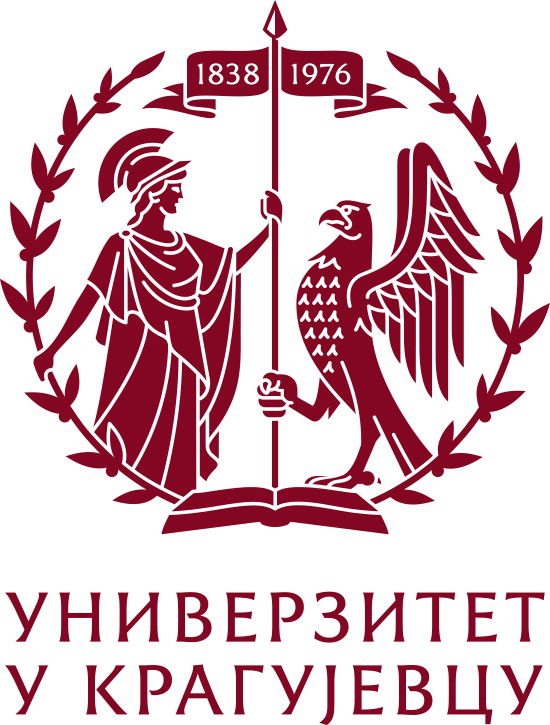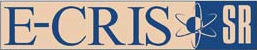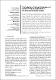Молимо вас користите овај идентификатор за цитирање или овај линк до ове ставке: `https://scidar.kg.ac.rs/handle/123456789/8936`
 Назив: The selection of optimal parameters of gerotor pump by application of factorial experimental design Аутори: Ivanović L.Gajević, SandraStojanovic, BlazaKandeva M.Jakimovska K. Датум издавања: 2017 Сажетак: © 2017, Faculty of Mechanical Engineering, Belgrade. The method of factorial experimental design for studying the factor influence on the flow rate and volumetric efficiency of the gerotor pump is applied in this paper. The considered factors are the coefficient of the trochoid radius, the number of revolution of a pump drive shaft and working pressure, where the first factor is a geometrical parameter and the others are working parameters. Factorial design and response surface methodology (RSM) are applied to evaluate and optimize the effects of these parameters on the flow rate and volumetric efficiency. The experimental design 33 is used, where each factor has three levels. Its optimal variant is obtained by the analysis of considered factors: the coefficient of the trochoid radius 1.375, the number of revolution of a pump drive shaft 2000 rpm and the working pressure 1 bar, where the the greatest values of flow rate and volumetric efficiency are obtained. A mathematical prediction model of the flow rate and volumetric efficiency has been developed in terms of above parameters. URI: https://scidar.kg.ac.rs/handle/123456789/8936 Тип: article DOI: 10.5937/fmet1701159I ISSN: 1451-2092 SCOPUS: 2-s2.0-85011411899 Налази се у колекцијама: Faculty of Engineering, Kragujevac66

#### Број преузимања

6

Датотеке у овој ставци:
Датотека Опис ВеличинаФормат
10.5937-fmet1701159I.pdf809.53 kBAdobe PDF